# Why understand how GHC works?

• Understand Core & STG – performance
• Familiarity with functional terminology
• Understand execution model – reasonable cost model

# The pipeline of GHC

Haskell -> GHC Haskell -> Core -> STG -> Cmm -> Assembly

• GHC Haskell: Used by libraries to implement Haskell proper but expose manual optimization opportunities and extract commonalities into library code rather than the compiler
• Core: ‘Simple’ functional language for optimization
• STG: Variant of core that makes laziness more explicit for easier compilation
• Cmm: Procedural language for portability among backends (LLVM or native-code-generator) and architectures (x86, ARM, PowerPC).

# GHC supports Haskell on top of an unsafe variant

Primitive types (GHC.Prim):

• Char#, Int#, Word#, Double#, Float#, Word#
• Array#, ByteArray#, ArrayArray#, MutableArray#
• State#

All primitive types are unlifted – can’t contain .

``ghci> :browse GHC.Prim``

# GHC supports Haskell on top of an unsafe variant

All variants of Int (In8, Int16, Int32, Int64) are represented internally by Int# (64bit) on a 64bit machine.

``````data Int32 = I32# Int# deriving (Eq, Ord, Typeable)

instance Num Int32 where
(I32# x#) + (I32# y#)  = I32# (narrow32Int# (x# +# y#))
...``````

Data constructors lift a type, allowing .

# GHC implements IO through the RealWorld token

• Trying to achieve: order of execution + execute once semantics
``````newtype IO a = IO (State# RealWorld -> (# State# RealWorld, a #))

returnIO :: a -> IO a
returnIO x = IO \$ \ s -> (# s, x #)

bindIO :: IO a -> (a -> IO b) -> IO b
bindIO (IO m) k = IO \$ \ s -> case m s of (# new_s, a #) -> unIO (k a) new_s``````
• `RealWorld` token enforces ordering through data dependence

# We’ve seen RealWorld Before

``````comp :: Handle -> IO ()
comp = do name <- hGetLine h
hPutStrLn h name``````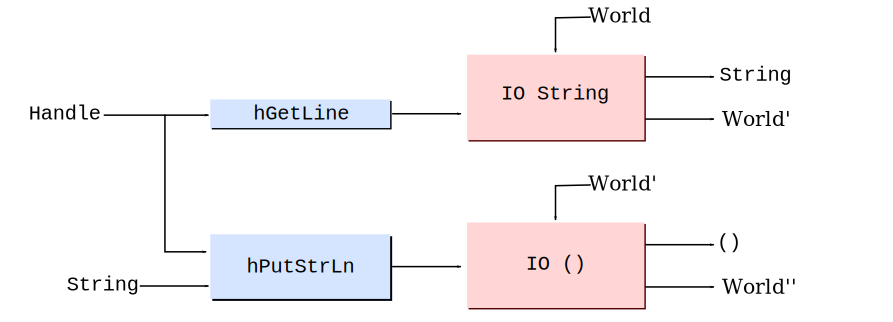``````comp :: GHC.IO.Handle.Types.Handle -> GHC.Prim.State# GHC.Prim.RealWorld
-> (# GHC.Prim.State# GHC.Prim.RealWorld, () #)
comp = \h rw1 ->
case GHC.IO.Handle.Text.hGetLine h rw1 of
(# rw2, str #) -> GHC.IO.Handle.Text.hPutStr h str rw2``````

# We implement unsafe IO operations by throwing away the world

• Various unsafe functions throw away `RealWorld` token
• No longer have guarantees about order or execution, or execute-one only semantics, optimizer could duplicate, or two threads could race and evaluate the same thunk
``````unsafePerformIO :: IO a -> a
unsafePerformIO m = unsafeDupablePerformIO (noDuplicate >> m)

unsafeDupablePerformIO  :: IO a -> a
unsafeDupablePerformIO (IO m) = lazy (case m realWorld# of (# _, r #) -> r)``````

# Core: a small function intermediate language

• Idea: map Haskell to a small lanuage for easier optimization and compilation

• Functional lazy language

• It consists of only a hand full of constructs!

``variables, literals, let, case, lambda abstraction, application``
• In general think, `let` means allocation, `case` means evaluation
``ghc -ddump-simpl M.hs > M.core``

# Core in one slide

``````data Expr b -- "b" for the type of binders,
= Var    Id
| Lit   Literal
| App   (Expr b) (Arg b)
| Lam   b (Expr b)
| Let   (Bind b) (Expr b)
| Case  (Expr b) b Type [Alt b]

| Type  Type
| Cast  (Expr b) Coercion
| Coercion Coercion

| Tick  (Tickish Id) (Expr b)

data Bind b = NonRec b (Expr b)
| Rec [(b, (Expr b))]

type Arg b = Expr b

type Alt b = (AltCon, [b], Expr b)

data AltCon = DataAlt DataCon | LitAlt  Literal | DEFAULT``````

Lets now look at how Haskell is compiled to Core.

# GHC Haskell to Core: monomorphic functions

``````idChar :: Char -> Char
idChar c = c``````

Core

``````idChar :: GHC.Types.Char -> GHC.Types.Char
[GblId, Arity=1]
idChar = \ (c :: GHC.Types.Char) -> c``````
• Functions are all lambda abstractions
• Names are fully qualified

# GHC Haskell to Core: polymorphic functions

``````id :: a -> a
id x = x

idChar2 :: Char -> Char
idChar2 = id``````

Core

``````id :: forall a. a -> a
id = \ (@ a) (x :: a) -> x

idChar2 :: GHC.Types.Char -> GHC.Types.Char
idChar2 = id @ GHC.Types.Char``````
• Types become arguments too! We explicitly pass types and instantiate polymorphic functions
• Type variables are proceeded by @ symbol (read them as ‘at type …’)
• This is known as second order lambda calculus
• GHC uses this representation as it helps with preserving type information during optimization

# GHC Haskell to Core: polymorphic functions

``````map :: (a -> b) -> [a] -> [b]
map _ []     = []
map f (x:xs) = f x : map f xs``````

Core

``````map :: forall a b. (a -> b) -> [a] -> [b]
map = \ (@ a) (@ b) (f :: a -> b) (xs :: [a]) ->
case xs of _
[]     -> GHC.Types.[] @ b
: y ys -> GHC.Types.: @ b (f y) (map @ a @ b f ys)``````
• Case statements are only place evaluation happens, read them as ‘evaluate’

New case syntax to make obvious that evaluation is happening:

``````case e of result
__DEFAULT -> result``````

# Where transformed to let

``````dox :: Int -> Int
dox n = x * x
where x = n + 2``````

Core

``````dox :: GHC.Types.Int -> GHC.Types.Int
dox = \ (n :: GHC.Types.Int) ->
let x :: GHC.Types.Int
x = GHC.base.plusInt n (GHC.Types.I# 2)
in GHC.base.multInt x x``````

# Patterns matching transformed to case statements

``````iff :: Bool -> a -> a -> a
iff True  x _ = x
iff False _ y = y``````

Core

``````iff :: forall a. GHC.Bool.Bool -> a -> a -> a
iff = \ (@ a) (d :: GHC.Bool.Bool) (x :: a) (y :: a) ->
case d of _
GHC.Bool.False -> y
GHC.Bool.True  -> x``````

# Type classes transformed to dictionaries

``````typeclass MyEnum a where
toId  :: a -> Int
fromId :: Int -> a``````

Core

``````data MyEnum a = DMyEnum (a -> Int) (Int -> a)

toId :: forall a. MyEnum a -> a -> GHC.Types.Int
toId = \ (@ a) (d :: MyEnum a) (x :: a) ->
case d of _
DMyEnum f1 _ -> f1 x

fromId :: forall a. MyEnum a -> GHC.Types.Int -> a
fromId = \ (@ a) (d :: MyEnum a) (x :: a) ->
case d of _
DMyEnum _ f2 -> f2 x``````
• Typeclasses are implemented via dictionary data type
• Functions that have type class constraints take an extra dictionary argument

# A dictionary constructed for each instance

``````instance MyEnum Int where
toId = id
fromId = id``````

Core

``````fMyEnumInt :: MyEnum GHC.Types.Int
fMyEnumInt =
DMyEnum @ GHC.Types.Int
(id @ GHC.Types.Int)
(id @ GHC.Types.Int)``````

# Dictionaries constructed from dictionaries

``````instance (MyEnum a) => MyEnum (Maybe a) where
toId (Nothing) = 0
toId (Just n)  = toId n
fromId 0       = Nothing
fromId n       = Just \$ fromId n``````

Core

``````fMyEnumMaybe :: forall a. MyEnum a -> MyEnum (Maybe a)
fMyEnumMaybe = \ (@ a) (dict :: MyEnum a) ->
DMyEnum @ (Maybe a)
(fMyEnumMaybe_ctoId @ a dict)
(fMyEnumMaybe_cfromId @ a dict)

fMyEnumMaybe_ctoId :: forall a. MyEnum a -> Maybe a -> Int
fMyEnumMaybe_ctoId = \ (@ a) (dict :: MyEnum a) (mx :: Maybe a) ->
case mx of _
Nothing -> I# 0
Just n  -> case (toId @ a dict n) of _ { I# y -> I# (1 +# y) }``````
• Function with `MyEnum (Maybe a)` constraint will take in a `MyEnum a` dictionary as an argument and call `fMyEnumMaybe` to construct the needed value.

# UNPACK unboxes types

``````data Point = Point {-# UNPACK #-} !Int
{-# UNPACK #-} !Int``````

Core

``data Point = Point Int# Int#``
• Only one data type for Point exists, GHC doesn’t duplicate it.

# UNPACK not always a good idea

``````addP :: P -> Int
addP (P x y ) = x + y``````

Core

``````addP :: P -> Int
addP = \ (p :: P) ->
case p of _
P x y -> case +# x y of z
__DEFAULT -> I# z``````
• Great code here as working with unboxed types

# UNPACK not always a good idea

``````module M where

add x y = x + y

module P where

Core

``````addP_bad = \ (p :: P) ->
case p of _
P x y ->
let x' = I# x
y' = I# y
• Need to unfortunately rebox the types since `add` only works with boxed types

# Core summary

• Look at Core to get an idea of how your code will perform
• Can see boxing and unboxing
• Language still lazy but `case` means evaluation, `let` means allocation

# Middle of GHC: Core -> Core

A lot of the optimizations that GHC does is through core to core transformations.

Lets look at two of them:

• Strictness and unboxing
• SpecConstr
``````Fun Fact: Estimated that functional languages gain 20 - 40%
improvement from inlining Vs. imperative languages which gain 10 - 15%``````

# Strictness & unboxing

Consider this factorial implementation in Haskell:

``````fac :: Int -> Int -> Int
fac x 0 = a
fac x n = fac (n*x) (n-1)``````
• In Haskell `x` & `n` must be represented by pointers to a possibly unevaluated objects (thunks)
• Even if evaluated still represented by “boxed” values on the heap

# Strictness & unboxing

Core

``````fac :: Int -> Int -> Int
fac = \ (x :: Int) (n :: Int) ->
case n of _
I# n# -> case n# of _
0#        -> x
__DEFAULT -> let one = I# 1
n' = n - one
x' = n * x
in  fac x' n'``````
• We allocate thunks before the recursive call and box arguments
• But `fac` will immediately evaluate the thunks and unbox the values!

# GHC with strictness analysis

Compile `fac` with optimizations.

``````\$wfac :: Int# -> Int# -> Int#
\$wfac = \ x# n# ->
case n# of _
0# -> x#
_  -> case (n# -# 1#) of n'#
_ -> case (n# *# x#) of x'#
_ -> \$wfac x'# n'#

fac :: Int -> Int -> Int
fac = \ a n ->
case a of
I# a# -> case n of
I# n# -> case (\$wfac a# n#) of
r# -> I# r#``````
• Create an optimized ‘worker’ and keep original function as ‘wrapper’ to preserve interface
• Must preserve semantics of `fac` `n = opt(fac)` `n`
• As the wrapper uses unboxed types and is tail recursive, this will compile to a tight loop in machine code!

# SpecConstr: Extending strictness analysis to paths

The idea of the SpecConstr pass is to extend the strictness and unboxing from before but to functions where arguments aren’t strict in every code path.

``````drop :: Int -> [a] -> [a]
drop n []     = []
drop 0 xs     = xs
drop n (x:xs) = drop (n-1) xs``````
• Not strict in first argument:
• `drop` [] = []
• `drop` (x:xs) =

# SpecConstr: Extending strictness analysis to paths

So we get this code without extra optimization:

``````drop n xs = case xs of
[]     -> []
(y:ys) -> case n of
I# n# -> case n# of
0 -> []
_ -> let n' = I# (n# -# 1#)
in drop n' ys``````
• But after the first call of drop, we are strict in `n` and always evaluate it!

# SpecConstr

The SpecConstr pass takes advantage of this to create a specialised version of `drop` that is only called after we have passed the first check.

``````-- works with unboxed n
drop' n# xs = case xs of
[]     -> []
(y:ys) -> case n# of
0# -> []
_  -> drop' (n# -# 1#) xs

drop n xs = case xs of
[]     -> []
(y:ys) -> case n of
I# n# -> case n# of
0 -> []
_ -> drop' (n# -# 1#) xs``````
• To stop code size blowing up, GHC limits the amount of specialized functions it creates (specified with the `-fspec-constr-threshol` and `-fspec-constr-count` flags)

# STG code

• After Core, GHC compiles to another intermediate language called STG

• STG is very similar to Core but has one nice additional property:
• laziness is ‘explicit’
• `case` = evaluation and ONLY place evaluation occurs (true in Core)
• `let` = allocation and ONLY place allocation occurs (not true in Core)
• So in STG we can explicitly see thunks being allocated for laziness using `let`
``ghc -ddump-stg A.hs > A.stg``

# STG code

``````map :: (a -> b) -> [a] -> [b]
map f []     = []
map f (x:xs) = f x : map f xs``````

STG

``````map :: forall a b. (a -> b) -> [a] -> [b]
map = \r [f xs]
case xs of _
[]     -> [] []
: z zs -> let bds = \u [] map f zs
bd  = \u [] f z
in : [bd bds]``````
• Lambda abstraction as `[arg1 arg2] f`
• `\r` - re-entrant function
• `\u` - updatable function (i.e., thunk)
• Data constructors applied with `[]`

# Graph reduction as a computational model for Haskell

Graph reduction is a good computational model for lazy functional languages.

``````f g = let x = 2 + 2
in (g x, x)``````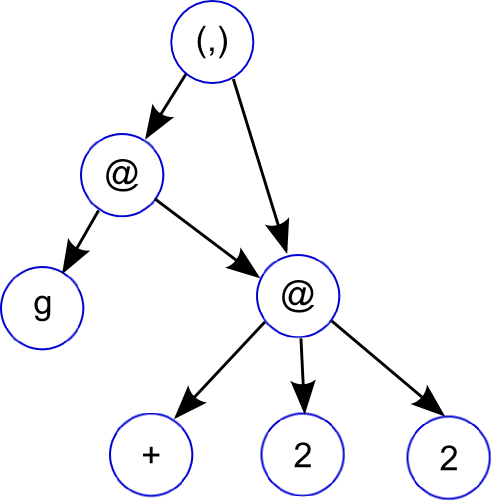# Graph reduction as a computational model for Haskell

Graph reduction is a good computational model for lazy functional languages.

``````f g = let x = 2 + 2
in (g x, x)``````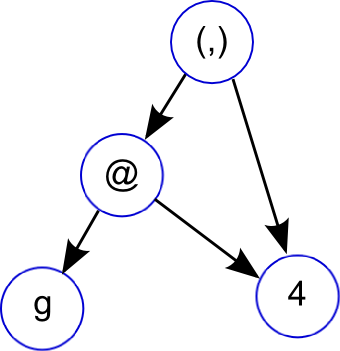# Graph reduction as a computational model for Haskell

Graph reduction is a good computational model for lazy functional languages.

• Graph reduction allows lazy evaluation and sharing
• let: adds new node to graph
• case: expression evaluation, causes the graph to be reduced
• When a node is reduced, it is replaced (or updated) with its result

Can think of your Haskell program as progressing by either adding new nodes to the graph or reducing existing ones.

# GHC execution model

• GHC uses closures as a unifying representation
• All objects in the heap are closures
• A stack frame is a closure

• GHC uses continuation-passing-style
• Functions will prepare stack in advance to setup call chains

# Closure representation

 Closure Info Table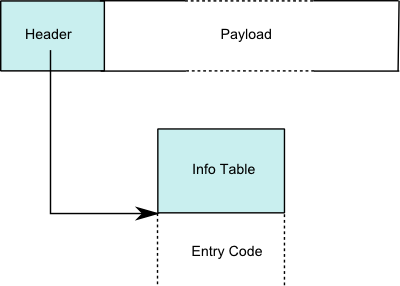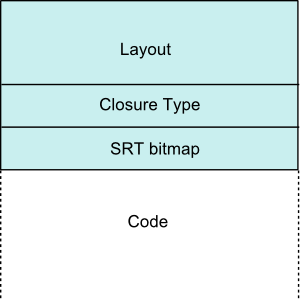• Header usually just a pointer to the code and metadata for the closure
• Get away with single pointer through positive and negative offsets
• Payload contains the closures environment (e.g free variables, function arguments)

# Data closure

``data G = G (Int -> Int) {-# UNPACK #-} !Int``
• `[Header | Pointers... | Non-pointers...]`
• Payload is the values for the constructor
• Entry code for a constructor just returns
``jmp Sp``

# Function closures

``````f = \x -> let g = \y -> x + y
in g x``````
• [Header | Pointers… | Non-pointers…]
• Payload is the bound free variables, e.g.,
• `[ &g | x ]`
• Entry code is the function code

# Partial application closures (PAP)

``foldr (:)``
• `[Header | Arity | Payload size | Function | Payload]`
• Arity of the PAP (function of arity 3 with 1 argument applied gives PAP of arity 2)
• Function is the closure of the function that has been partially applied
• PAPs should never be entered so the entry code is some failure code

# Thunk closures

``range = [1..100]``
• `[Header | Pointers... | Non-pointers...]`
• Payload contains the free variables of the expression
• Differ from function closure in that they can be updated
• Entry code is the code for the expression

# Calling convention

• On X86 32bit - all arguments passed on stack
• On X86 64bit - first 5 arguments passed in registers, rest on stack

• `R1` register in Cmm code usually is a pointer to the current closure (i.e., similar to `this` in OO languages)

• Thunks once evaluated should update their node in the graph to be the computed value
• GHC uses a self-updating-model – code unconditionally jumps to a thunk. Up to thunk to update itself, replacing code with value
• If thunk already evaluated, then entry code just returns``````mk :: Int -> Int
mk x = x + 1``````
``````// thunk entry - setup stack, evaluate x
mk_entry()
entry:
if (Sp - 24 < SpLim) goto gc;       // check for enough stack space

I64[Sp - 16] = stg_upd_frame_info;  // setup update frame (closure type)
I64[Sp -  8] = R1;                  // set thunk to be updated (payload)

I64[Sp - 24] = mk_exit;             // setup continuation (+)

Sp = Sp - 24;                       // decrease stack
R1 = I64[R1 + 8];                   // grab 'x' from environment
jump I64[R1] ();                    // eval 'x'

gc: jump stg_gc_enter_1 ();``````
• `stg_upd_frame_info` RTS function that handles updating a thunk with it’s result.

``````mk :: Int -> Int
mk x = x + 1``````
``````// thunk exit - setup value on heap, tear-down stack
mk_exit()
entry:
Hp = Hp + 16;
if (Hp > HpLim) goto gc;

v::I64 = I64[R1] + 1;               // perform ('x' + 1)

I64[Hp - 8] = GHC_Types_I_con_info; // setup Int closure
I64[Hp + 0] = v::I64;

R1 = Hp;                            // point R1 to computed thunk value
Sp = Sp + 8;                        // pop stack

gc: HpAlloc = 16;
jump stg_gc_enter_1 ();``````

# stg_upd_frame_info code updates a thunk with its value

• To update a thunk with its value we need to change its header pointer
• Should point to code that simply returns now
• Payload also now needs to include the value

• Naive solution would be to synchronize on every thunk access
• But we don’t need to! Races on thunks are fine since we can rely on purity Races just leads to duplication of work
• This is one reason why `unsafeDupablePerformIO` can lead duplication! And explains the check that `unsafePerformIO` has to avoid this

# stg_upd_frame_info code updates a thunk with its value

Thunk closure:

• `[Header | Pointers... | Non-pointers...]`

• `Header` = `[ Info Table Pointer | Result Slot ]`

• Result slot empty when thunk unevaluated

• Update code first places result in result slot and secondly changes the info table pointer

• Safe to do without synchronization (need write barrier) on all architectures GHC supports: no thread will see the new info table pointer without a valid result slot pointer

# Avoiding entering values with pointer tagging

• Evaluation model is we always enter a closure, even values

• This is poor for performance, we prefer to avoid entering values every single time

• An optimization that GHC does is pointer tagging. The trick is to use the final bits of a pointer which are usually zero (last 2 for 32bit, 3 on 64) for storing a ‘tag’

• GHC uses this tag for:
• If the object is a constructor, the tag contains the constructor number (if it fits)
• If the object is a function, the tag contains the arity of the function (if it fits)

# Avoiding entering values

Our example code from before:

``````mk :: Int -> Int
mk x = x + 1``````

Changes with pointer tagging:

``````mk_entry()
entry:
...
R1 = I64[R1 + 16];          // grab 'x' from environment
if (R1 & 7 != 0) goto cxd;  // check if 'x' is eval'd
jump I64[R1] ();            // not eval'd so eval
cxd: jump mk_exit ();            // 'x' eval'd so jump to (+) continuation
}

mk_exit()
cx0:
I64[Hp - 8] = ghczmprim_GHCziTypes_Izh_con_info; // setup Int closure
I64[Hp + 0] = v::I64;               // setup Int closure
R1 = Hp - 7;                        // point R1 to computed thunk value (with tag)
...
}``````

# Pointer tagging makes your own data types efficient

• If the closure is a constructor, the tag contains the constructor number (if it fits).
``data MyBool a = MTrue a | MFalse a``
• Will be as efficient as using an `Int#` for representing true and false.

• If your type has more constructors than the tag bits allow (4 or more on 32bit, 8 or more on 64bit) then GHC just uses the tag bits 0 or 1 to represent evaluated or unevaluated.

# Pointer tagging avoids looking up the info table

``````mycase :: Maybe Int -> Int
mycase x = case x of Just z -> z; Nothing -> 10``````

Cmm

``````mycase_entry()                          // corresponds to forcing 'x'
entry:
R1 = R2;                        // R1 = 'x'
I64[Sp - 8] = mycase_exit;      // setup case continuation
Sp = Sp - 8;
if (R1 & 7 != 0) goto crL;      // check pointer tag to see if x eval'd
jump I64[R1] ();                // x not eval'd, so eval
exit:

mycase_exit()                           // case continuation
entry:
v::I64 = R1 & 7;                // get tag bits of 'x' and put in local variable 'v'
if (_crD::I64 >= 2) goto crE;   // can use tag bits to check which constructor we have
R1 = stg_INTLIKE_closure+417;   // 'Nothing' case
Sp = Sp + 8;
exit:
R1 = I64[R1 + 6];               // get 'z' thunk inside Just
Sp = Sp + 8;
R1 = R1 & (-8);                 // clear tags on 'z'
jump I64[R1] ();                // force 'z' thunk``````

# Bringing it all home

No lecture on Compilers is complete without assembly code!

``````add :: Int -> Int -> Int
add x y = x + y + 2``````
``````A_add_info:
.LcvZ:
leaq -16(%rbp),%rax
cmpq %r15,%rax
jb .Lcw1
movq %rsi,-8(%rbp)
movq %r14,%rbx
movq \$sul_info,-16(%rbp)
testq \$7,%rbx
jne sul_info
jmp *(%rbx)
.Lcw1:
jmp *-8(%r13)

sul_info:
.LcvS:
movq 8(%rbp),%rax
movq 7(%rbx),%rcx
movq %rcx,8(%rbp)
movq %rax,%rbx
movq \$suk_info,0(%rbp)
testq \$7,%rbx
jne suk_info
jmp *(%rbx)

suk_info:
.LcvK:
cmpq 144(%r13),%r12
ja .LcvP
movq 7(%rbx),%rax
movq 8(%rbp),%rcx
movq \$ghczmprim_GHCziTypes_Izh_con_info,-8(%r12)
movq %rcx,0(%r12)
leaq -7(%r12),%rbx
jmp *0(%rbp)
.LcvP:
movq \$16,184(%r13)
.LcvQ:
jmp *-16(%r13)``````

# Finished!

• So that’s is all I can cover in this lecture.

• I haven’t covered a few significant areas:
• Typechecking
• Garbage collection
• The scheduler: threads, multi-processor support
• Foreign Function Interface
• Profiling
• Infrastructure of the compiler: Interface files, packages, modular compilation… ect
• Final code generators
• GHCi
• The finer details of lazy evaluation: blackholes

# Resources & References

Here are some resources to learn about GHC, they were also used to create these slides: